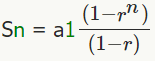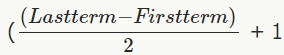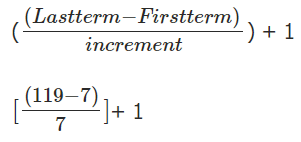GMAT  >  Sequences and Series

# Sequences and Series - Notes | Study Integrated Reasoning for GMAT - GMAT

 Table of contentsGeometric sequence vs arithmetic sequenceSequence vs SeriesSum of odd numbers in a series1 Crore+ students have signed up on EduRev. Have you?

There are two types of sequences and two types of series. They are geometric sequences and arithmetic sequences, and geometric series and arithmetic series.

Geometric sequence vs arithmetic sequence

An arithmetic sequence is a sequence of numbers where each new term after the first is formed by adding a fixed amount called the common difference to the previous term in the sequence.
Set A = {1,2,3,4,5,6,7,8,9,10}
Set B = {2,4,6,8,10,12,14}
Set C ={3,8,13,18,23,28}
In 'set A', the common difference is the fixed amount of one. In 'set B' the common difference is the fixed amount of two, and in 'set C' the common difference is the fixed amount of five. As you most likely noticed already, the common difference is found by finding the difference between two consecutive terms within the sequence. For example, in 'set C', to find the common difference compute (8 - 3 = 5).
A geometric sequence on the other hand, is a sequence of numbers where each term after the first is found by multiplying the previous term by a fixed number called the common ratio.
Set D={2,4,8,16,32}
Set E={3,9,27,81}
Set F={5,10,20,40,80}
You might notice that the difference between consecutive numbers in the above three sets are not a fixed amount. For instance, in 'set F', the first two terms (5 and 10) have a smaller difference than the last two terms (40 and 80). Therefore, the above sets are geometric sequences. The difference between two consecutive numbers is therefor the common ratio. To find the common ratio you simply take the ratio one consecutive number to the one before it. In 'set F' this would be (10/5=2). Therefore, n 'set F' the common ratio is two. In 'set E' the common ratio is (27/9=3). In 'set' D' the common ratio is two (32/16=2).
Summary
The difference between the two types of sequences is that in arithmetic sequences the consecutive numbers in a set differ by a fixed amount known as the common difference whereas in a geometric sequence the consecutive numbers in a set differ by a fixed number known as the common ratio.

Sequence vs Series

This is quite simple. A sequence is a list of numbers. A series is created by adding terms in the sequence. There you go, now you know the difference. So if you take 'set A' and add the terms then you have an arithmetic series. If you take 'set D' and add the terms, then you have a geometric series.
Sequence: {1, 3, 5, 7, 9, …}
Series: {1+3+5+7+9+…}

What the GMAT could ask us to do with sequences and series and how to do it!
There is no limit to what the GMAT can ask you to find when dealing with series and sequences. Here are some examples of things you may be asked to find/do with them.
(1) The sum of numbers in a series (which can be asked in many tricky ways such as the sum of all the numbers, sum of just the even numbers, sum of just the odd numbers, sum of only the numbers which are multiples of 7, sum of the first 10 numbers, and many more tricky ways!)
(2) The nth term in a sequence
(3) How many integers are there in a sequence
Anyway, now that you get the point... lets give you the formulas that will allow you to answer any question regarding series and sequences. I will then show you how to use the formulas to answer some questions that might not be intuitive of non math geniuses.

Formula for geometric sequence (when there is a common ratio)

dark green means subscript
Recursive (to find just the next term):
an = an-1 * r
Explicit (to find any nth term):
an = a1 * rn−1rn−1
an = nth term
a1 = the first term
r = common ratio
In reality, you only need to know the explicit formula, because you can find any term with it. I only put the recursive formula for understanding.

Formula for arithmetic sequence (when there is a common difference)

dark green means subscriptSn = Sum of first nth terms

a1 = first term

r = common ratio

n = nth term

Formula for arithmetic series (when there is a common ratio)

dark green means subscript

Sn = n/2(a1 + an)
Sn = n/2(First term + Last term)
The above two equations are the same (I put them in both ways because some prep programs teach "first + last" but it is important to see that in the first of the two, the last term is identified as an. Well what if you do not know the last term? Then you have to calculate it using the equation for the nth term (solving for an) of an arithmetic sequence which is listed above... or you can substitute the formula for an into the first one of these two by replacing an with what is equals and simplifying. You get the following:
Sn = n/2[2a + (n-1)d]
Sn = sum of the series
a1 = the first term
an = the nth term
n = the number of terms
d = the common difference
PART 2 ----------------------------
Now that we know all this information, there are some important things that are understood as well to ensure that the formulas are used correctly.

How to find the number of integers in a set

(Last term - First term) + 1 *A mistake is that people will forget to add the 1. The number of terms between 3 and 10 is not 7, it is 8. A common mistake is that people will calculate (10-3=7)... but this is wrong. Remember, as Manhattan GMAT says, "Add one before you are done". *Notice how I used the word "term" and not number. This is important because sometimes you don't always just put the first and last number you are given. For example, If you are asked to find the number of even integers between 1 and 30, you don't use the "first number" in the set. The first number is "1", which is odd, and we are only speaking about even numbers. Therefore, the first term is "2", not "1", even though the set or question might have stated "from 1-30". Same goes with the last term. There is another step needed to answer this question though.

Find number of odd integers (or even) in a set*If the question is to find the number of odd integers between 2 and 30, then your first term is 3, and your last term is 29. They must be odd to fit in the set you are asked to analyze. *If the question is find the number of even integers between 3 and 29, then your first term is 4, and your last term is 28.

Find number of integers that are a multiple of a certain number in a set

GMAT questions can get tricky, but luckily not too tricky. For example... What if you are asked to "find the number of multiples of 7 between 2 and 120"?All you have to do is instead of dividing our old formula by 2, you divide it by the increment. Also, notice how my first and last terms are the first term that is a multiple of 7 and the last term that is a multiple of seven within the set!

Sum of odd numbers in a series

This seems to be a popular topic on GMAT forums. Its quite simple. You already know everything you need to after reading this post. It is a two step problem. Here are the two steps:
(1) Find the number of odd terms. This is you "n" value now.
(2) Plug in the "n" value into the formula for an arithmetic series.
PART III
(1) The mean and the medium of any arithmetic sequence is equal to the average of the first and last terms.
(2) The sum of an arithmetic sequence is equal to the mean (average) times the number of terms.
(3) The product of n consecutive integers is always divisible by n! So, 4x5x6 (4*5*6=120) is divisible by 3!
(4) If you have an odd number of terms in consecutive set, the sum of those numbers is divisible by the number of terms.
(5) number four (above) does not hold true for consecutive sets with an even amount of terms.

The document Sequences and Series - Notes | Study Integrated Reasoning for GMAT - GMAT is a part of the GMAT Course Integrated Reasoning for GMAT.
All you need of GMAT at this link: GMAT

## Integrated Reasoning for GMAT

24 videos|33 docs|24 tests
 Use Code STAYHOME200 and get INR 200 additional OFF

## Integrated Reasoning for GMAT

24 videos|33 docs|24 tests

Track your progress, build streaks, highlight & save important lessons and more!

,

,

,

,

,

,

,

,

,

,

,

,

,

,

,

,

,

,

,

,

,

;